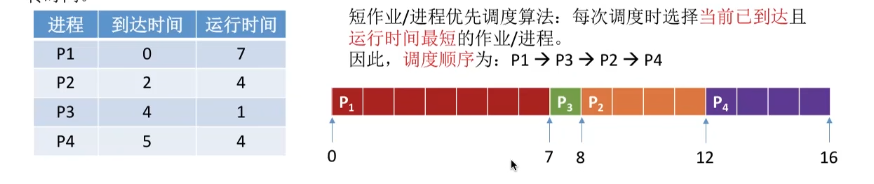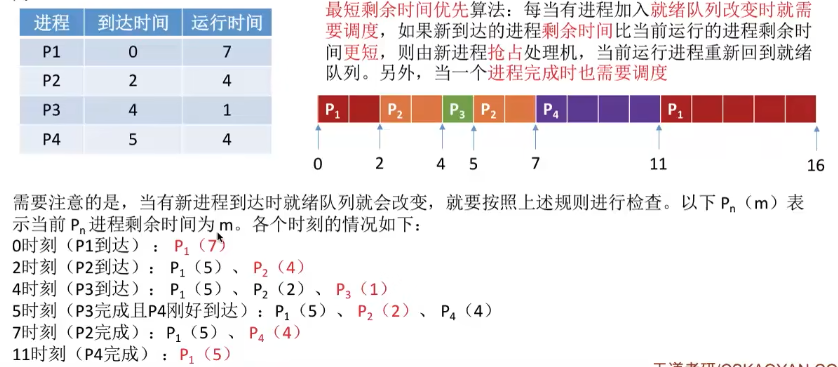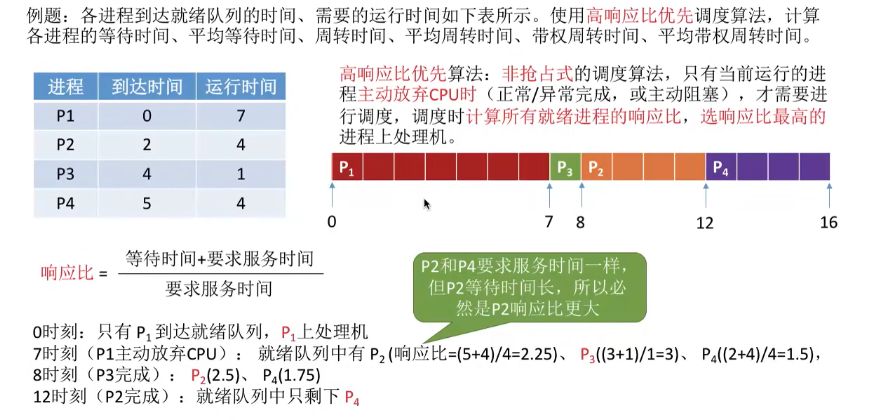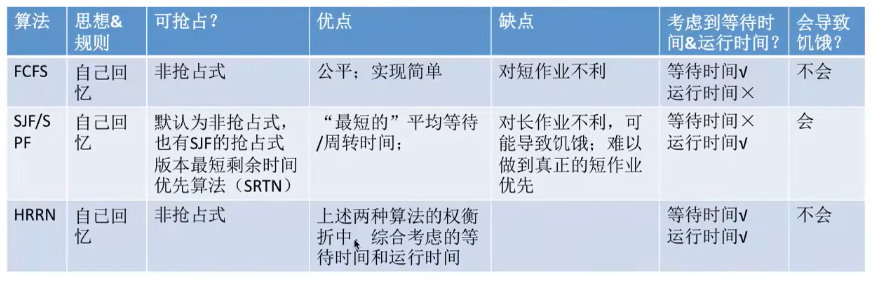# 只言片语

:D 获取中...

#### 1.先来先服务（FCFS，First Come Firse Serve）

##### FCFS
• 算法思想：主要从“公平”的角度考虑（类似于生活中排队买东西）

• 算法规则：按照作业/进程到达的先后顺序进行服务

• 用于作业/进程调度：用于作业调度时，考虑的是哪个作业先到达后备队列；用于进程调度时，考虑的是那个进程先到达就绪队列

• 是否可抢占？ 非抢占式的算法

• 优缺点：

• 优点：公平、算法实现简单
• 缺点：排在长作业（进程）后面的短作业需要等待很长时间，带权周转时间很大，对作业来说用户体验不好。即：FCFS算法对长作业有利，对短作业不利（EG:排队买奶茶）
• 是否会导致饥饿（某进程，作业长期得不到服务）：不会（只要进程或作业一直等着总会得到服务）• 周转时间 = 完成时间 - 到达时间

1. P1 = 7 - 0 = 7
2. P2 = 11 - 2 = 9
3. P3 = 12 - 4 = 8
4. P4 = 16 - 5 = 11
• 带权周转时间 = 周转时间 / 运行时间

1. P1 = 7 / 7 = 1
2. P2 = 9 / 4 = 2.25
3. P3 = 8 / 1 = 8
4. P4 = 11 / 4 = 2.75
• 等待时间 = 周转时间 - 运行时间

1. P1 = 7 - 7 = 0
2. P2 = 9 - 7 = 2
3. P3 = 8 - 1 = 8
4. P4 = 11 - 4 = 7
• 平均周转时间

• （7 + 9 + 8 + 11）/ 4 = 8.75
• 平均带权周转时间

• （1 + 2.25 + 8 + 2.75）/ 4 = 3.5

• 平均等待时间

• （0 + 5 + 7 + 7）/ 4 = 4.75

#### 2.短作业优先（SJF，Shortest Job First）

##### SJF
• 算法思想：追求最少的平均等待时间，最少的平均周转时间，最少的平均平均带权周转时间

• 算法规则：最短作业/进程得到优先服务（所谓“最短”，是指要求服务时间最短）

• 用于短作业.进程调度：既可用于作业调度，也可用于进程调度。用于进程调度时成为“短进程优先（SPF，Shortest Process First）”

• 是否可抢占？：SJF和SPF是非抢占式算法，但是也有抢占式的版本——最短剩余时间优先算法（SRTF，Shortest Remaining Time Next）

• 优缺点：

• 优点：“最短的”平均等待时间，平均周转时间
• 缺点：不公平。对短作业有利，对长作业不利。可能产生饥饿现象。另外，作业/进程的运行时间是由用户提供的，不一定真实，不一定能做到真正的短作业优先。
• 是否会导致饥饿：会。如果有源源不断的短作业/进程进来，可能会使长作业/进程长时间得不到服务，产生“饥饿”现象。如果一直得不到服务，则成为**”饿死”**。• 周转时间 = 完成时间 - 到达时间

1. P1 = 7 - 0 = 7
2. P3 = 8 - 4 = 4
3. P2 = 12 - 2 = 10
4. P4 = 16 - 5 = 11
• 带权周转时间 = 周转时间 / 运行时间

1. P1 = 7 / 7 = 1
2. P3 = 4 / 1 = 4
3. P2 = 10 / 4 = 2.5
4. P4 = 11 / 4 = 2.75
• 等待时间 = 周转时间 - 运行时间

1. P1 = 7 - 7 = 0
2. P3 = 4 - 1 = 3
3. P2 = 10 - 4 = 6
4. P4 = 11 - 5 = 6
• 平均周转时间

• （7 + 4 + 10 + 11）/ 4 = 8
• 平均带权周转时间

• （1 + 4 + 2.5 + 2.75）/ 4 = 2.56

• 平均等待时间

• （0 + 3 + 6 + 7）/ 4 = 4• 周转时间 = 完成时间 - 到达时间

1. P1 = 16 - 0 = 16
2. P2 = 7 - 2 = 5
3. P3 = 5 - 4 = 1
4. P4 = 11 - 5 = 6
• 带权周转时间 = 周转时间 / 运行时间

1. P1 = 16 / 7 = 2.28
2. P2 = 5 / 4 = 1.25
3. P3 = 1 / 1 = 1
4. P4 = 6 / 4 = 1.5
• 等待时间 = 周转时间 - 运行时间

1. P1 = 16 - 7 = 9
2. P2 = 5 - 4 = 1
3. P3 = 1 - 1 = 0
4. P4 = 6 - 4 = 2
• 平均周转时间

• （16 + 5 + 1 + 6）/ 4 = 7
• 平均带权周转时间

• （2.28 + 1.25 + 1 + 1.5）/ 4 = 1.50

• 平均等待时间

• （9 + 1 + 0 + 2）/ 4 = 3

1. 如果题目中未特别说明，所提到的“短作业/短进程优先算法”默认非抢占式的
2. 很多书上都会说“SJF调度算法的平均等待时间、平均周转时间最少”

1. 穗盐染个来说SJF的平均等待时间，平均周转时间并不一定最少，但是相比于其他算法（如FCFS），SJF依然可以获得较少的平均等待时间、平均周转时间
2. 如果选择题中遇到“SJF算法的平均等待时间，平均周转时间最少”的选项，那最好判断其它选项是不是有很明显的错误，如果没有合适的选项，那应该选择该项。

#### 3.高响应比优先算法（HRRN，Highest Response Ratio Next）

##### 对FCFS和SJF两种算法的思考
• FCFS算法是在每次调度的时候选择一个等待时间最长的作业（进程）为其服务。但是没有考虑到作业的运行时间，因此导致了对短作业不友好的问题。

• SJF算法是选择一个执行时间最短的作业为其服务。但是又完全不考虑各个作业的等待时间，因此导致了对长作业不友好的问题，甚至还会造成界问题

• 能不能设计一个算法，既考虑到各个作业的等待时间，也能兼顾运行时间呢？

高响应比优先算法

（老折中了）

##### HRRN
• 算法思想：要综合考虑作业/进程的等待时间和要求服务时间

• 算法规则：在每次调度时先计算各个作业/进程的响应比，选择响应比最高的作业/进程为其服务。

$响应比=\frac{等待时间+要求服务时间}{要求服务时间}$，在这里响应比肯定是会>=1 的

• 用于作业/进程调度：既可用于作业调度，也可用于进程调度

• 是否可抢占？：非抢占式的算法，因此只有当前运行的作业/进程主动放弃处理及时，才需要调度，才需要计算响应比

• 优缺点：

• 优点：综合考虑了等待时间和运行时间（要求服务时间）。等待时间相同时，要求服务时间短的优先（SJF的优点）。要求服务时间相同时，等待时间长的优先（FCFS的优点）。对于长作业来说，随着等待时间越来越久，其相应比也会越来越大，从而避免了长作业饥饿的问题

• 是否会导致饥饿：不会#### 4.知识回顾